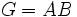Maximal factorization

Contents

This article is about a definition in group theory that is standard among the group theory community (or sub-community that dabbles in such things) but is not very basic or common for people outside.
VIEW: Definitions built on this | Facts about this: (facts closely related to Maximal factorization, all facts related to Maximal factorization) |Survey articles about this | Survey articles about definitions built on this
VIEW RELATED: Analogues of this | Variations of this | Opposites of this |
View a list of other standard non-basic definitions

Definition

Symbol-free definition

A maximal factorization of a group is an expression of the group as the product of two maximal subgroups.

Definition with symbols

A maximal factorization of a group$G$ is an expression$G = AB$ where both$A$ and$B$ are maximal subgroups of$G$.